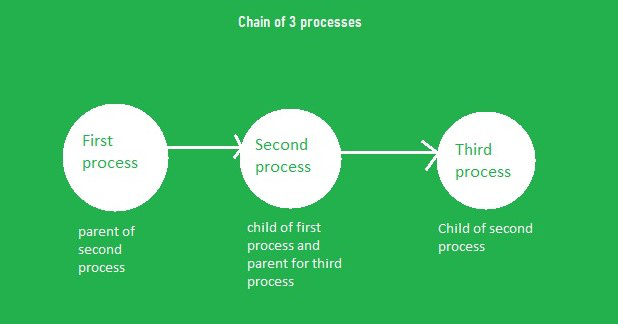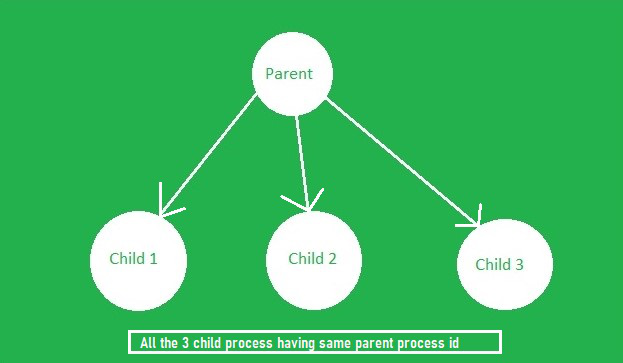# Chain processes vs Fan of processes using fork() function in C

Fork System Call: The fork system call is used for creating a new process, which is called the child process, which runs concurrently with the process that makes the fork() call (parent process). After a new child process is created, both processes will execute the next instruction following the fork() system call. A child process uses the same program counter, same CPU registers, the same open files which are used in the parent process.

For creating a fan or chain of processes, first, insert the header file “unistd.h” to use the fork() function for creating the process. For using the exit() method include “stdlib.h” and there are 3 ways exit statement can be used in the program:

• exit(0): For normal termination
• exit(1): For abnormal termination
• exit(): It can be normal and abnormal.

### Chain Of Process:

Suppose there are three processes, and the first process is the parent process, and it is creating the child process, then this second process creates another process (third process), then the first process becomes the parent of the second one and the second process becomes the parent of the third process. So, a chain of processes is obtained, and that’s called a chain of processes.Chain of 3 processes

Below is the implementation to create a chain of processes using the fork():

## C

 `// C program to create a chain of process ` `#include ` `#include ` `#include ` `#include ` `#include ` ` `  `// Driver Code ` `int` `main() ` `{ ` `    ``int` `pid; ` ` `  `    ``// Iterate in the range [0, 2] ` `    ``for` `(``int` `i = 0; i < 3; i++) { ` `        ``pid = fork(); ` ` `  `        ``if` `(pid > 0) { ` `            ``// Print the parent process ` `            ``printf``(``"Parent process ID is %d\n"``, ` `                   ``getpid()); ` `            ``break``; ` `        ``} ` `        ``else` `{ ` `            ``// Print the child process ` `            ``printf``(``"Child process ID is %d\n"``, ` `                   ``getpid()); ` `        ``} ` `    ``} ` ` `  `    ``return` `0; ` `} `

Output

```Parent process ID is 1359
Child process ID is 1360
Parent process ID is 1360
Child process ID is 1360
Child process ID is 1361
Parent process ID is 1361
Child process ID is 1360
Child process ID is 1361
Child process ID is 1362```

Explanation: In the above program, getpid() is used to get the process ID. Alternately, getppid() can also be used which will get a parent process ID. Each time when the program is run, the output is different, because not every time the same process id is allocated to the process by the operating system, but the PID(process id) is always obtained in non-decreasing order whenever the program is run.

### Fan Of Process:

Suppose there are three processes, and the first process is the parent process, and it is creating two child processes. If both of the processes have the same parent, then it is a fan of processes.Fan of 3 processes

Below is the implementation to create a fan of processes using fork():

## C

 `// C program to create a fan of processes ` ` `  `#include ` `#include ` `#include ` `#include ` ` `  `// Driver Code ` `int` `main() ` `{ ` `    ``// Iterate in the range [0, 2] ` `    ``for` `(``int` `i = 0; i < 3; i++) { ` `        ``if` `(fork() == 0) { ` `            ``// getpid gives process id ` `            ``// getppid gives parent process id ` `            ``printf``(``"child pid %d from the"` `                   ``" parent pid %d\n"``, ` `                   ``getpid(), getppid()); ` ` `  `            ``// Set Normal termination of ` `            ``// the program ` `            ``exit``(0); ` `        ``} ` `    ``} ` ` `  `    ``for` `(``int` `i = 0; i < 3; i++) ` `        ``wait(NULL); ` `} `

Output

```child pid 29831 from parent pid 29830
child pid 29832 from parent pid 29830
child pid 29833 from parent pid 29830```

Explanation: In the above program, every time the same process ID is not allocated every time to process by the operating system. That means in this case, also the output is different each time the program is run, but the child processes created will have the same parent process ID.

Unlock the Power of Placement Preparation!
Feeling lost in OS, DBMS, CN, SQL, and DSA chaos? Our Complete Interview Preparation Course is the ultimate guide to conquer placements. Trusted by over 100,000+ geeks, this course is your roadmap to interview triumph.